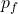## If an individual has a momentum of 700 kgm/s (forward) and collides with an individual with a momentum of 400 kgm/s in the opposing directio

Question

If an individual has a momentum of 700 kgm/s (forward) and collides with an individual with a momentum of 400 kgm/s in the opposing direction (backward), what is the resulting velocity of the collusion if the first person weighs 50kg and the 2nd person weighs 60kg?

in progress 0
6 months 2021-08-25T19:06:20+00:00 2 Answers 6 views 0

## Answers ( )

v = 2.72 m / s

Explanation:

Let’s write the equation of the moment

p = mv

In this case the system is formed by the two people

Initial moment

p₀ = p₁ – p₂

Final moment= (m₁ + m₂) v

p₀ = p_{f}

p₁ – p₂ = (m₁ + m₂) v

v = (p₁ -p₂) / (m₁ + m₂)

Let’s calculate

v = (700 – 400) / (50 + 60)

v = 2.72 m / s

The resulting velocity of collision is 2.72m/s.

Explanation:

Total momentum before collision= Momentum of individual A + Momentum of individual B

Momentum of individual A = 700 kgm/s (forward)

Momentum of individual B(backward) = Because the momentum of individual B is in the opposing direction , the momentum of individual B will carrying a negative sign = -400kgm/s

Total momentum before collision = 700kgm/s + ( -400kgm/s)

= 700kgm/s – 400kgm/s

= 300kgm/s.

After the collision of the two individuals, the mass of the two individuals would become one.

Mass of Individual A = 50kg

Mass of Individual B = 60kg

Total mass= Mass A + Mass B

= 50 kg + 60kg

= 110kg

The law of linear momentum states that if no external force acts on the system of two colliding objects or individuals, the sum of the linear momentum of both objects remains the same.

This law above applies to this question , therefore the Total momentum before the collision is equal to the total momentum after collision.

Therefore the Total momentum after collision is 300kgm/s

To find the resulting velocity after collision:

Total momentum after collision = Total mass × Resulting velocity after collision.

Resulting velocity after collision = Total momentum after collision ÷ Total mass of the two individuals

= 300kgm/s ÷ 110kg

Resulting velocity after collision = 2.72m/s